# RD Sharma Solutions for Class 11 Maths Exercise 21.2 Chapter 21 - Some Special Series

Sometimes the nth term of a series cannot be determined by the methods discussed so far, if a series is such that the difference between the successive terms is either in A.P. or G.P. then we can make use of the ‘method of difference’ concept. Students who face difficulty in scoring marks in Maths subjects are advised to refer to RD Sharma Solutions. RD Sharma Class 11 Maths Solutions is the best reference material any student can use to secure their desired scores in the board exams. The links to the solutions of this exercise can be accessed in the RD Sharma Class 11 Maths pdf, which can be downloaded easily from the links given below.

## Download the pdf of RD Sharma Solutions for Class 11 Maths Exercise 21.2 Chapter 21 – Some Special Series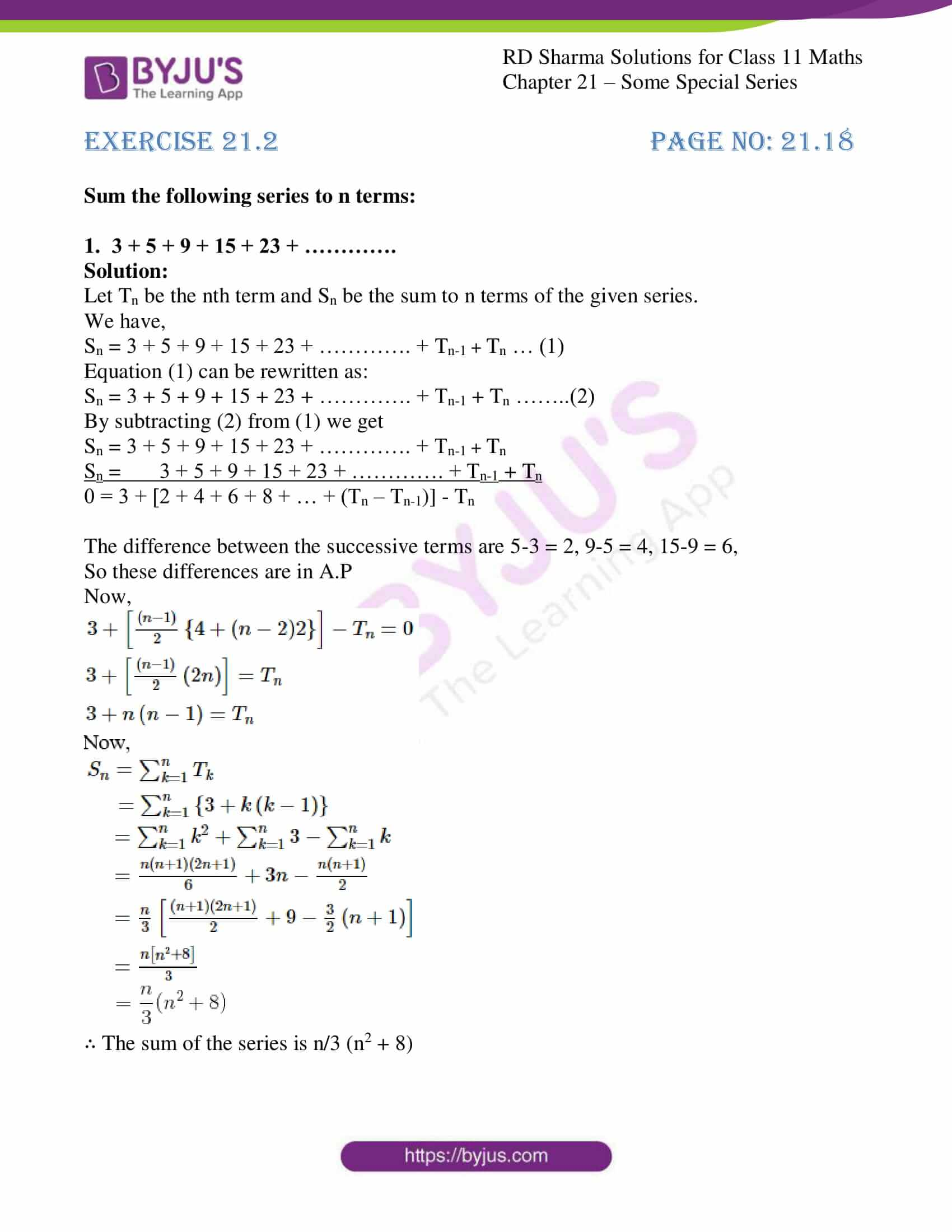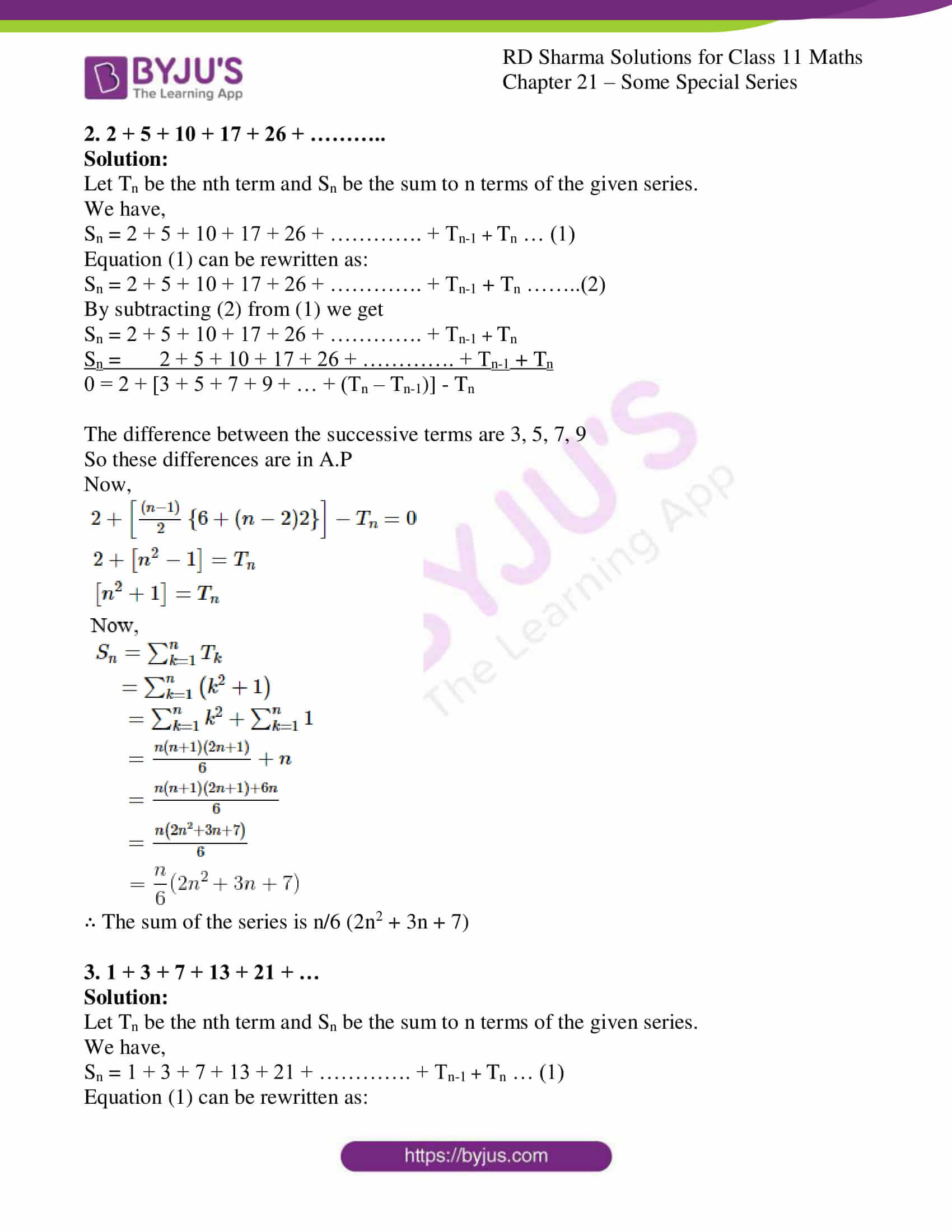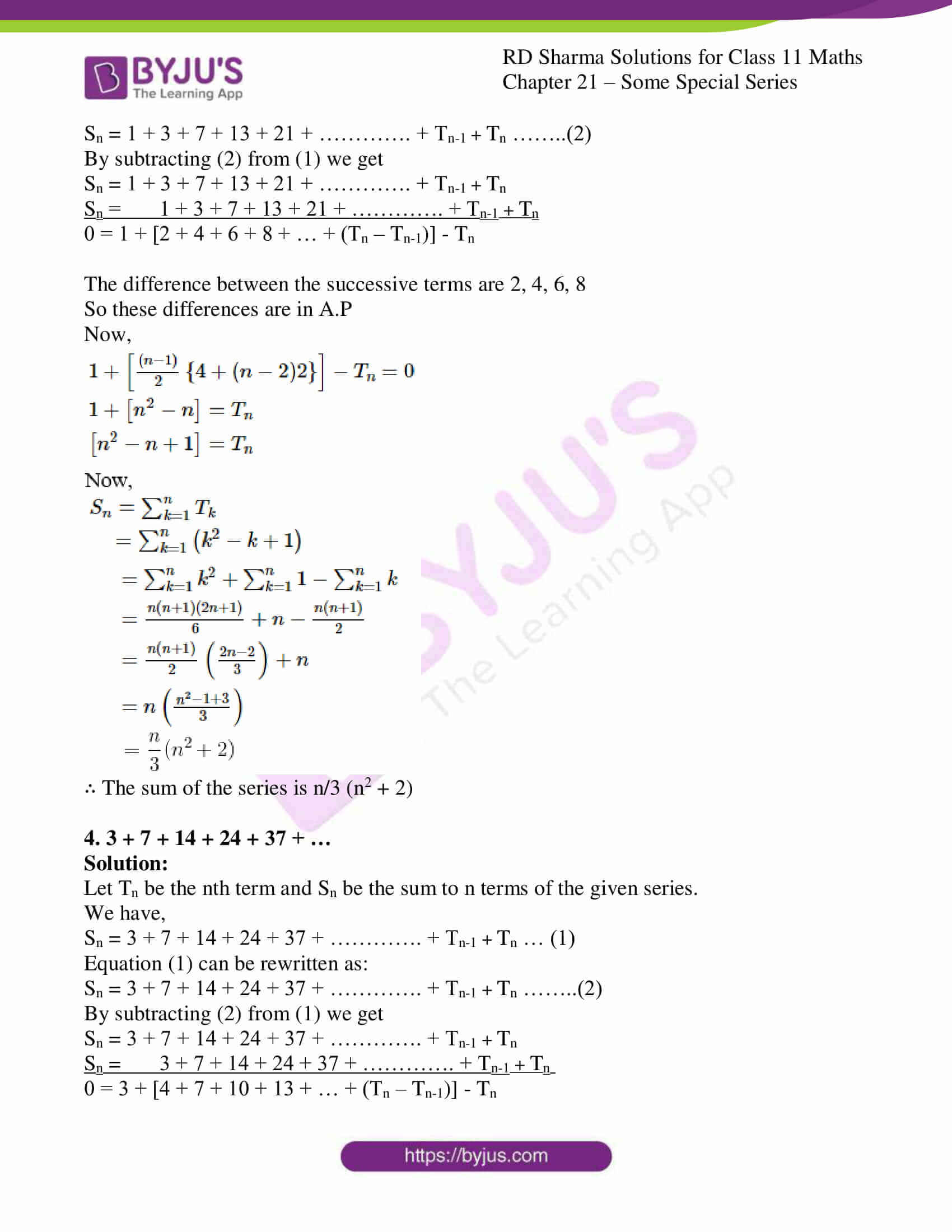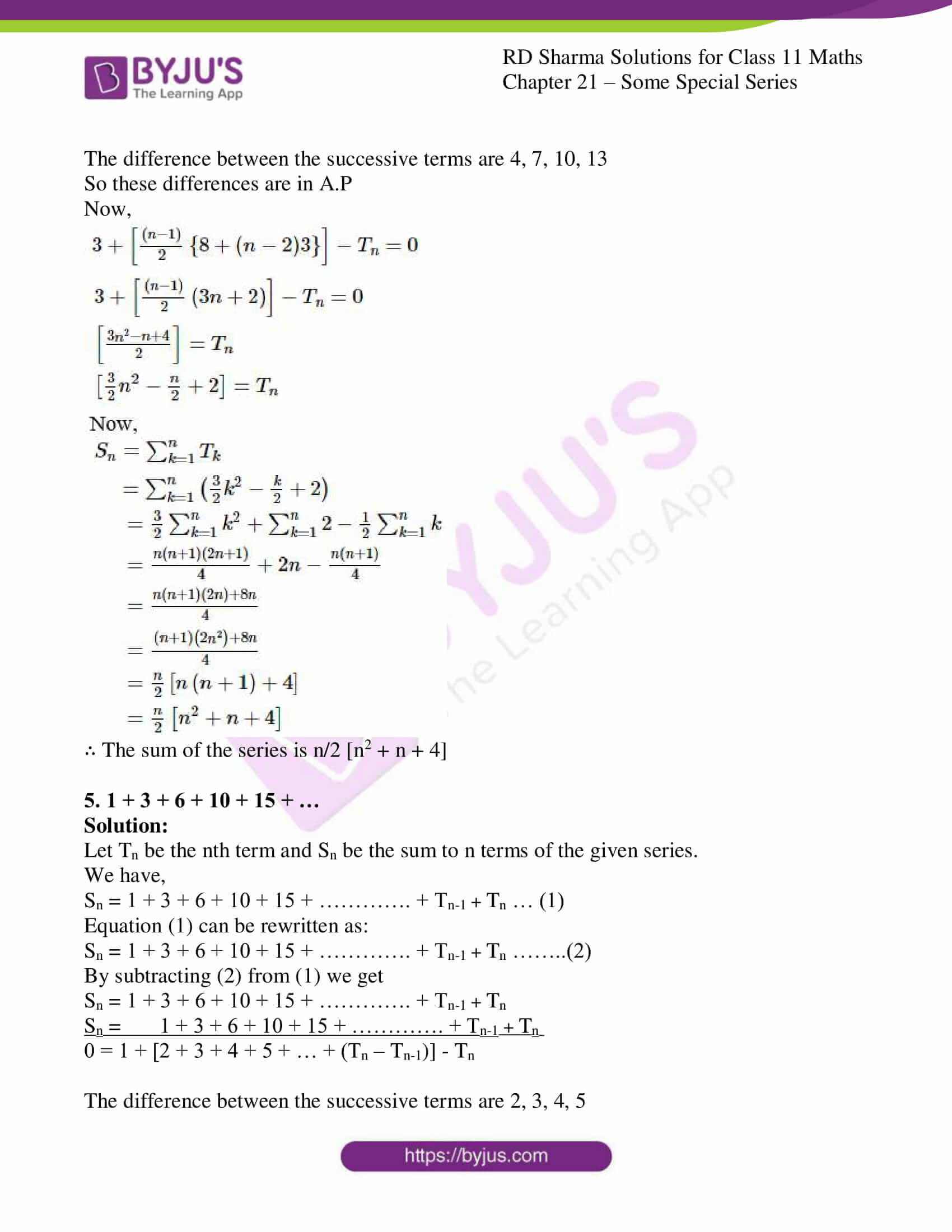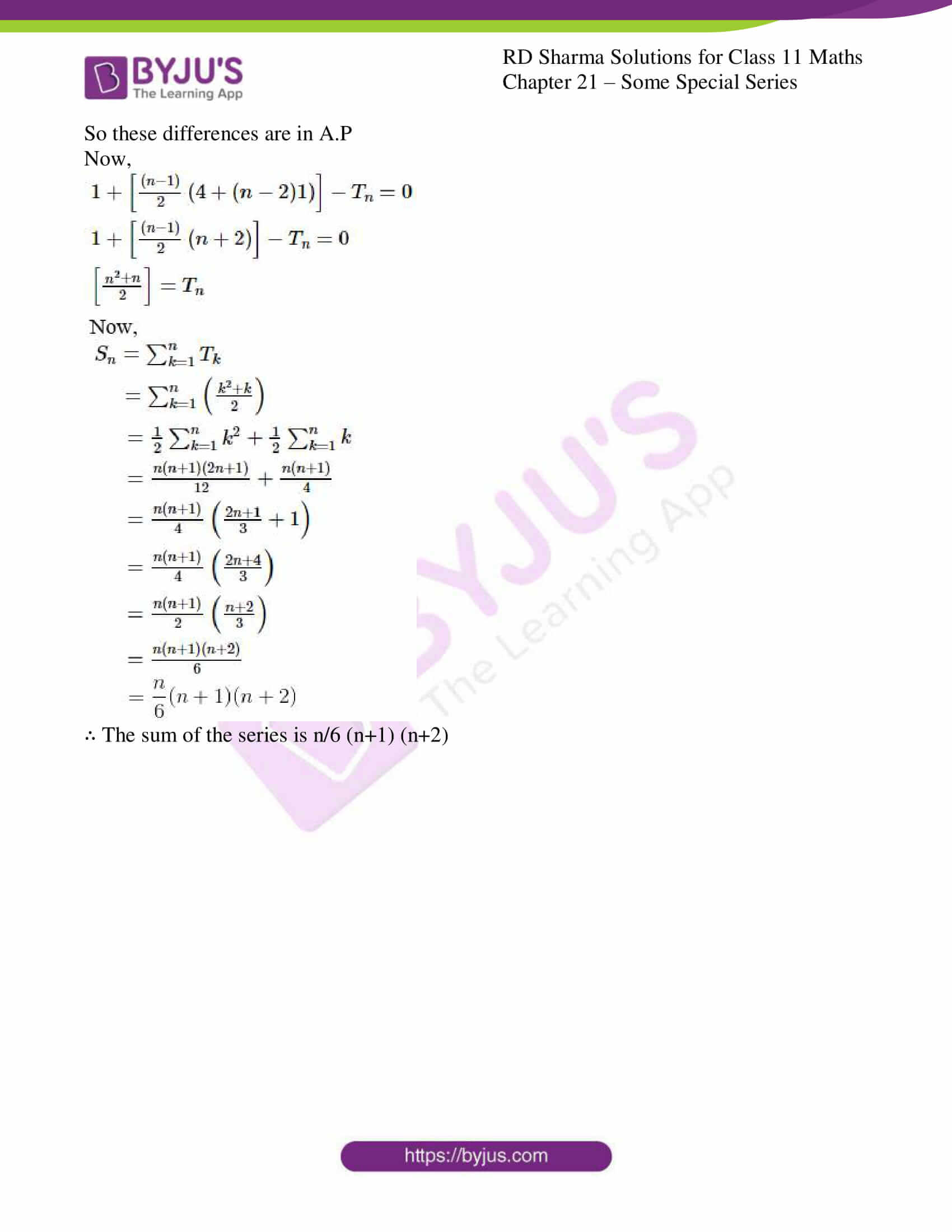### Access answers to RD Sharma Solutions for Class 11 Maths Exercise 21.2 Chapter 21 – Some Special Series

Sum the following series to n terms:

1. 3 + 5 + 9 + 15 + 23 + ………….

Solution:

Let Tn be the nth term and Sn be the sum to n terms of the given series.

We have,

Sn = 3 + 5 + 9 + 15 + 23 + …………. + Tn-1 + Tn … (1)

Equation (1) can be rewritten as:

Sn = 3 + 5 + 9 + 15 + 23 + …………. + Tn-1 + Tn ……..(2)

By subtracting (2) from (1) we get

Sn = 3 + 5 + 9 + 15 + 23 + …………. + Tn-1 + Tn

Sn = 3 + 5 + 9 + 15 + 23 + …………. + Tn-1 + Tn

0 = 3 + [2 + 4 + 6 + 8 + … + (Tn – Tn-1)] – Tn

The difference between the successive terms are 5-3 = 2, 9-5 = 4, 15-9 = 6,

So these differences are in A.P

Now,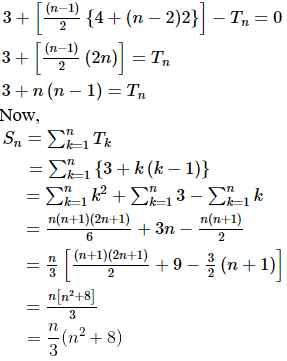∴ The sum of the series is n/3 (n2 + 8)

2. 2 + 5 + 10 + 17 + 26 + ………..

Solution:

Let Tn be the nth term and Sn be the sum to n terms of the given series.

We have,

Sn = 2 + 5 + 10 + 17 + 26 + …………. + Tn-1 + Tn … (1)

Equation (1) can be rewritten as:

Sn = 2 + 5 + 10 + 17 + 26 + …………. + Tn-1 + Tn ……..(2)

By subtracting (2) from (1) we get

Sn = 2 + 5 + 10 + 17 + 26 + …………. + Tn-1 + Tn

Sn = 2 + 5 + 10 + 17 + 26 + …………. + Tn-1 + Tn

0 = 2 + [3 + 5 + 7 + 9 + … + (Tn – Tn-1)] – Tn

The difference between the successive terms are 3, 5, 7, 9

So these differences are in A.P

Now,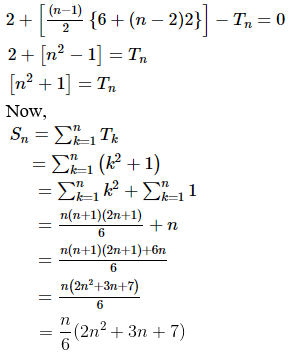∴ The sum of the series is n/6 (2n2 + 3n + 7)

3. 1 + 3 + 7 + 13 + 21 + …

Solution:

Let Tn be the nth term and Sn be the sum to n terms of the given series.

We have,

Sn = 1 + 3 + 7 + 13 + 21 + …………. + Tn-1 + Tn … (1)

Equation (1) can be rewritten as:

Sn = 1 + 3 + 7 + 13 + 21 + …………. + Tn-1 + Tn ……..(2)

By subtracting (2) from (1) we get

Sn = 1 + 3 + 7 + 13 + 21 + …………. + Tn-1 + Tn

Sn = 1 + 3 + 7 + 13 + 21 + …………. + Tn-1 + Tn

0 = 1 + [2 + 4 + 6 + 8 + … + (Tn – Tn-1)] – Tn

The difference between the successive terms are 2, 4, 6, 8

So these differences are in A.P

Now,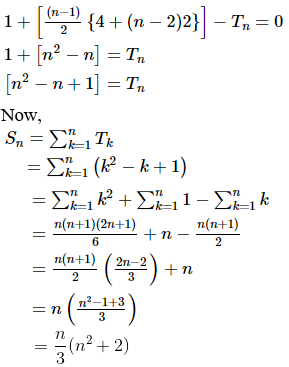∴ The sum of the series is n/3 (n2 + 2)

4. 3 + 7 + 14 + 24 + 37 + …

Solution:

Let Tn be the nth term and Sn be the sum to n terms of the given series.

We have,

Sn = 3 + 7 + 14 + 24 + 37 + …………. + Tn-1 + Tn … (1)

Equation (1) can be rewritten as:

Sn = 3 + 7 + 14 + 24 + 37 + …………. + Tn-1 + Tn ……..(2)

By subtracting (2) from (1) we get

Sn = 3 + 7 + 14 + 24 + 37 + …………. + Tn-1 + Tn

Sn = 3 + 7 + 14 + 24 + 37 + …………. + Tn-1 + Tn

0 = 3 + [4 + 7 + 10 + 13 + … + (Tn – Tn-1)] – Tn

The difference between the successive terms are 4, 7, 10, 13

So these differences are in A.P

Now,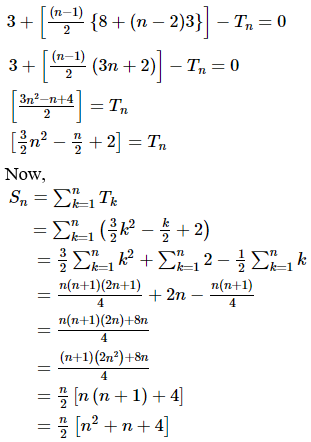∴ The sum of the series is n/2 [n2 + n + 4]

5. 1 + 3 + 6 + 10 + 15 + …

Solution:

Let Tn be the nth term and Sn be the sum to n terms of the given series.

We have,

Sn = 1 + 3 + 6 + 10 + 15 + …………. + Tn-1 + Tn … (1)

Equation (1) can be rewritten as:

Sn = 1 + 3 + 6 + 10 + 15 + …………. + Tn-1 + Tn ……..(2)

By subtracting (2) from (1) we get

Sn = 1 + 3 + 6 + 10 + 15 + …………. + Tn-1 + Tn

Sn = 1 + 3 + 6 + 10 + 15 + …………. + Tn-1 + Tn

0 = 1 + [2 + 3 + 4 + 5 + … + (Tn – Tn-1)] – Tn

The difference between the successive terms are 2, 3, 4, 5

So these differences are in A.P

Now,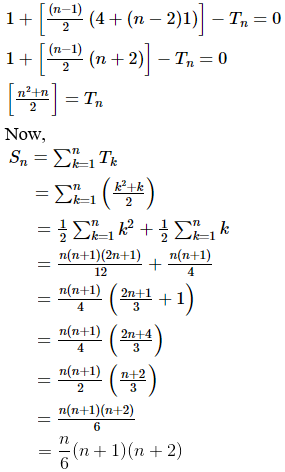∴ The sum of the series is n/6 (n+1) (n+2)